Signal Processing > Laplace Transform PairsShowing results 1 to 10 of 10, on page 1 of 2
 DESCRIPTION EQUATION Laplace transform involving the unit step function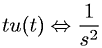Laplace transform involving the unit step functionLaplace transform involving the unit step function and an exponentialLaplace transform involving the unit step function and an exponential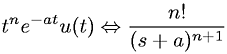Laplace transform involving the unit step function and cosine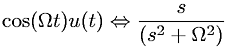Laplace transform involving the unit step function and sine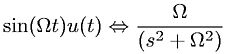Laplace transform involving the unit step function, cosine, and an exponential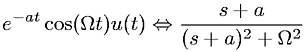Laplace transform involving the unit step function, sine, and an exponential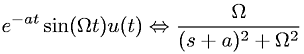Laplace transform of Kroeneker delta functionLaplace transform of unit step function times a constant (K)